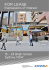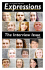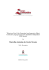# 9th Grade | Unit 1

## Transcription

9th Grade | Unit 1
```MATH
STUDENT BOOK
9th Grade | Unit 1
Unit 1 | Variables and Numbers
Math 901
Variables and Numbers
INTRODUCTION |3
1.EXPRESSIONS
5
VARIABLES |5
NUMBER SKILLS |18
THE DISTRIBUTIVE PROPERTY |23
SELF TEST 1 |33
2. SIGNED NUMBERS
37
DEFINITION |37
SUBTRACTION |45
MULTIPLICATION |49
DIVISION |52
SELF TEST 2 |55
GLOSSARY |58
LIFEPAC Test is located in the
center of the booklet. Please
remove before starting the unit.
Section 1 |1
Variables and Numbers | Unit 1
Author: Robert L. Zenor, M.A., M.S.
Editor:
Richard W. Wheeler, M.A.Ed.
Consulting Editor:
Rudolph Moore, Ph.D.
Revision Editor:
Alan Christopherson, M.S.
Westover Studios Design Team:
Phillip Pettet, Creative Lead
Teresa Davis, DTP Lead
Nick Castro
Andi Graham
Jerry Wingo
804 N. 2nd Ave. E.
Rock Rapids, IA 51246-1759
© MCMXCVI by Alpha Omega Publications, Inc. All
rights reserved. LIFEPAC is a registered trademark of
Alpha Omega Publications, Inc.
All trademarks and/or service marks referenced in this material are the property of their respective
owners. Alpha Omega Publications, Inc. makes no claim of ownership to any trademarks and/
or service marks other than their own and their affiliates, and makes no claim of affiliation to any
companies whose trademarks may be listed in this material, other than their own.
Some clip art images used in this curriculum are from Corel Corporation, 1600 Carling Avenue, Ottawa,
Ontario, Canada K1Z 8R7. These images are specifically for viewing purposes only, to enhance the
presentation of this educational material. Any duplication, resyndication, or redistribution for any
other purpose is strictly prohibited. Other images in this unit are © 2009 JupiterImages Corporation
2| Section 1
Unit 1 | Variables and Numbers
Variables and Numbers
INTRODUCTION
This LIFEPAC® is your introduction to a system of mathematics unlike the arithmetic you learned in the elementary grades. In arithmetic you were taught the
rules that govern the four operations of the system—addition, subtraction,
multiplication, and division; and you were told which operation to perform on a
given set of numbers to get the answer. Here are typical examples of exercises in
arithmetic: 4 + 3 = 7, 7 – 2 = 5, 13 x 4 = 52, 12 ÷ 3 = 4.
Algebra, like geometry, trigonometry, and calculus, is another of several mathematical systems. Like arithmetic, it has its own operating rules. Unlike arithmetic, algebra often requires you to find the value of one of the numbers—the
unknown—in an exercise. Sometimes, you will have to decide for yourself what
operation to use, and sometimes several operations will be used. Toward the
end of this LIFEPAC, you will learn how to apply the arithmetic operations to
numbers less than zero—the negative numbers.
Objectives
Read these objectives. The objectives tell you what you will be able to do when
you have successfully completed this LIFEPAC. When you have finished this
LIFEPAC, you should be able to:
1. Identify bases, exponents, constants, variables, numerical coefficients,
terms, sums, and products.
2. Simplify algebraic expressions when possible.
3. Evaluate algebraic expressions.
4. Translate algebraic expressions.
5. Perform operations with signed numbers.
Section 1 |3
Variables and Numbers | Unit 1
________________________________________________________________________________________________ ________________________________________________________________________________________________
________________________________________________________________________________________________
________________________________________________________________________________________________
________________________________________________________________________________________________
________________________________________________________________________________________________
________________________________________________________________________________________________
________________________________________________________________________________________________
________________________________________________________________________________________________
________________________________________________________________________________________________
________________________________________________________________________________________________
________________________________________________________________________________________________
________________________________________________________________________________________________
________________________________________________________________________________________________
________________________________________________________________________________________________
________________________________________________________________________________________________
________________________________________________________________________________________________
________________________________________________________________________________________________
________________________________________________________________________________________________
4| Section 1
Unit 1 | Variables and Numbers
1.EXPRESSIONS
The expression 8 + 3 is a numerical
expression: numerical because it consists of
numbers; expression because it expresses
an operation, in this case addition.
In algebra letters of the alphabet are
used to represent numbers. These letters
are referred to either as unknowns or as
variables. An expression that contains
a variable, such as n + 3, is an algebraic
expression. Learning to handle algebraic
expressions is the first step in this new
system of mathematics. You will have an
opportunity in this section to review and
practice basic number skills and then to
apply those skills in simplifying expressions
by the distributive property.
OBJECTIVES
Review these objectives. When you have completed this section, you should be able to:
1. Identify bases, exponents, constants, variables, numerical coefficients, terms, sums,
and products.
2. Simplify algebraic expressions when possible.
3. Evaluate algebraic expressions.
4. Translate algebraic expressions.
VARIABLES
If expressions, whether numerical or
algebraic, imply addition, they are called
sums; if they imply subtraction, they are
called differences; if multiplication, products;
and if division, quotients. These four
operations will now be used in evaluating
expressions.
SUMS AND DIFFERENCES
In the expression n + 3, n and 3 are
addends. Since we have inserted the plus
sign between the letter n and the number
3, the expression is called an indicated sum.
Its value cannot be determined until we
know the value of n.
means that n is to be subtracted from
3. n – 3 is called an indicated difference.
The expressions n – 3 and 3 – n are not
necessarily equal, because subtraction is an
ordered operation. We see that 8 – 3 cannot
be 3 – 8. The differences are different.
In an algebraic expression, the letter that
represents a number is called a variable. In
the expression n + 8, n is the variable and 8
is the constant.
Here are some other models of sums and
differences.
5 – y, x + 6, A + 10, A + B, x + y, x – y
The expression n – 3 means that 3 is to
be subtracted from n. Likewise, 3 – n
Section 1 |5
Variables and Numbers | Unit 1
Simplify. Work from left to right and perform any operation in parentheses first.
Model:
9 + 12 – 3 = 21 – 3 = 18
(2 + 5) – 4 = 7 – 4 = 3
1.1
9+6
1.2
8 + 13
1.3
5 + 22
1.4
17 + 16
1.5
32 + 43
1.6
9+5+4
1.7
3+8+4
1.8
10 + 15 + 4
1.9
17 + 18 + 5
1.10
14 + 13 + 7
1.11
10 – 6 + 8
1.12
15 – 4 + 1
1.13
17 – 3 – 4
1.14
13 – 8 + 10
1.15
28 + 4 – 10
1.16
5 + (6 – 4)
1.17
10 + (3 – 2)
1.18
29 – (7 + 2)
1.19
(13 + 2) – 8
1.20
(50 + 5) – 11
Write the meaning of each of the following expressions.
Model: x + 10
1.21
n+5
1.22
n– 5
1.23
x+8
1.24
x–8
6| Section 1
The sum of some number x and 10.
Unit 1 | Variables and Numbers
1.25
8–x
1.26
5–y
1.27
x + (5 + 7)
1.28
x – (8 + 2)
1.29
x + (8 – 2)
1.30
x+x
Identify the variable and constant in each of the following expressions and tell if it
is a sum, a difference, or neither.
Variable
Constant
b.
8
Operation
Model:
x–8
a.
1.31
6+y
a.
b.
c.
1.32
N–8
a.
b.
c.
1.33
A
a.
b.
c.
1.34
B–3
a.
b.
c.
1.35
C + 10 + 12
a.
b.
c.
x
c.difference
Write an algebraic expression of each of the following statements.
1.36
The sum of n and 6.
1.37
The difference of 8 and n.
1.38
The difference of n and 10.
1.39
The sum of n and itself.
1.40
The sum of n and the sum of 8 and 6.
Section 1 |7
Variables and Numbers | Unit 1
Sums like 8 + 3 may be written as 3 + 8.
The sum 11 is the same in either case. The
ability to interchange addends is called the
commutative property of addition.
Also, sums like 4 + 2 + 7 may be obtained
from (4 + 2) + 7 or from 4 + (2 + 7).
Model:
The sum 13 is the same in either case.
The ability to change the grouping of the
addends is called the associative property of
These two properties can be used to
simplify expressions.
S implify 3 + x + 7.
3+x+7=x +3+7
= x + (3 + 7)
= x + 10
Simplify
1.41
x+7+8
1.42
7+x+3
1.43
9+7+n
1.44
x + 15 – 4
1.45
(20 + 2) + r
1.46
8+r– 4
1.47
15 + x + 10 – 4
1.48
(15 – 10) + n
1.49
5 + n + (15 – 2)
1.50
1.5 + 3.82
1.51
17.25 + 3.9
1.52
19.62 + 8.33 + 5.7
1.53
1.005 + 3.54
1.54
73.05 + 8.006
1.55
15.63 + 7.956 + 82.735
1.56
25.63 – 8.23
1.57
73.543 – 23.683
1.58
28.543 – 14.26 – 3.65
8| Section 1
1.55
15.63 + 7.956 + 82.735
1.56
25.63 – 8.23Unit 1 | Variables and Numbers
1.57
73.543 – 23.683
1.58
28.543 – 14.26 – 3.65
1.59
x + 6.2 + 8.5
1.60
7.5 + n + 9.63
1.61
81.56 + n – 2.55
1.62
7.95 – 3.86 + N
1.63
22.6 + x – 11.3 + 1.2
1.64
77.65 – 15.56 + x + 1.2
PRODUCTS
The numerical expression 7 + 7 can be
renamed several ways, one of which is 2
times 7. We wish to omit the (x) as a times
sign. In algebra we will use the dot, 2 • 7,
or the parentheses, (2)(7). Therefore, the
product of 6 and 9 will be written as 6 • 9
or (6)(9). Likewise, if one of the factors is
literal — the n in 7 times n — we will write
the product as 7n. The dot or parentheses
are not to be used when writing literal
products.
 Models:
6 • 4, 6x, 5n, 15r, r17, A15
Product expressions such as r • 17 and A
• 15 are to be written with the constant
preceding the variable, 17r and 15A.
The constant preceding the variable in a
product is called a numerical coefficient.
Section 1 |9
Variables and Numbers | Unit 1
Find the product of each of the following expressions.
1.65
6•5
1.66
8•4
1.67
7•6
1.68
9•8
1.69
4 • 10
1.70
(5)(10)
1.71
(15)(12)
1.72
(3)(50)
1.73
(40)(5)
1.74
(70)(20)
1.75
6(15)
1.76
7(22)
1.77
8(15)
1.78
16(30)
1.79
10(23)
1.80
5•7•8
1.81
12 • 5 • 8
1.82
3(4)(5)
1.83
6(2)(5)
1.84
15(2)(8)
Name the numerical coefficient of each of the following expressions.
1.85
6x
1.86
5n
1.87
22r
1.88
16p
1.89
13q
1.90
8 • 2N
1.91
3 • 2x
1.92
7.2r
1.93
9(14)P
1.94
2(3)(6)q
10| Section 1
Unit 1 | Variables and Numbers
In the operation 5 • 7, the product is
the same if the expression is changed
to 7 • 5. That is, 5 • 7 = 7 • 5. The ability
to interchange factors is called the
commutative property of multiplication. Also,
the associative property of multiplication
allows you to change the grouping of the
factors.
These two properties can be used to
simplify expressions. When more than
one variable is used, the letters are to be
written in alphabetical order.
Model 1:
Rewrite B • 5 • A
B •5•A = 5•B •A
= 5 • (B • A )
= 5 • (A • B)
= 5A B
Model 2:
Rewrite 7 • K • 5 • H
7 • K • 5 • H may be rewritten as
(7 • 5)(H • K ) using the commutative
and associative properties; thus,
the simplified form is 35HK .
Simplify. Remember: When more than one variable is used, the letters are to be
written in alphabetical order. Also, no dots are to be shown in the final answers.
1.95
6•x•7
1.96
5•P•2
1.97
3•S•R
1.98
8•x•2•y
1.99
a• c • 2 • 5
1.100
C •5•2•A
1.101
4•Q•2•P
1.102
10 • K • 2
Section 1 |11
Variables and Numbers | Unit 1
Write the meaning of each of the following expressions.
Model:
4A
Model:
10N – 2
1.103
7n
1.104
6P
1.105
8N + 5
1.106
7 + 2x
1.107
12x – 10
1.108
52 – 25x
The product of 4 and some number.
The difference between ten times some number and 2.
EXPONENTS
The numerical expression 5 times 5 may be
written as 52. The 2 is called an exponent.
The exponent is a counter for the number
of repeated factors.
Thus 6 • 6 = 62 and 8 • 8 • 8 = 83.
Models: x2 = x • x
x3 = x • x • x
x4 = x • x • x • x
In the case of literal expressions, we have
x • x = x2 and A • A • A = A3. Conversely, x3
means x • x • x, or three factors of x.
P2 = P • P
P3 = P • P • P
P4 = P • P • P • P
(ab) 2 = ab • ab
(ab) 3 = ab • ab • ab
(ab) 4 = ab • ab • ab • ab
x2 is read, “The square of x” or “x squared.”
x3 is read, “The cube of x” or “x cubed.”
x4 is read, “The fourth power of x” or “x to the fourth.”
xn is an indicated power. x is called the base,
and n is the exponent of the base.
12| Section 1
Unit 1 | Variables and Numbers
In each case, identify the base and the exponent of the indicated power.
Model: 38
base = 3
Base
exponent = 8
E xponent
Base
1.109
26
1.110
39
1.111
510
1.112
83
1.113
x6
1.114
y5
1.115
7n
1.116
9P
1.117
15 x + 1
1.118
103x–1
E xponent
Write each of the following expressions in product form.
Model: A 3 = A • A • A
1.119
63
=
1.120
74
=
1.121
x2
=
1.122
y5
=
1.123
33
=
1.124
14
=
1.125
25
=
1.125
( 2 )3
=
1.127
(2.5) 2
=
1.128
(.01) 4
=
1
Section 1 |13
Variables and Numbers | Unit 1
Simplify each of the following expressions.
Model: 32 = 3 • 3 = 9
1.129
23
1.130
42
1.131
53
1.132
35
1.133
103
1.134
104
1.135
172
1.136
202
1.137
73
Circle the larger number in each pair.
Model: 12, 22 since 12 = 1 • 1 = 1 and 22 = 2 • 2 = 4
1.138
25 or 52
1.139
52 or 53
1.140
32 or 23
1.141
33 or 25
1.142
( 2 ) 3 or ( 3 ) 2
1.143
(.2) 2 or (.3) 2
1
1
Write the following products in exponential form.
Model:
A • A • A = A3
1.144
x• x• x• x
1.145
B• B• B• B• B
1.146
P•P
1.147
N•N•N
1.148
A • B• A • B
1.149
C • d• C • d• C
1.150
xyyx
1.151
PPQQQ
1.152
abcabcabc
1.153
xyzxyz
14| Section 1
Unit 1 | Variables and Numbers
EVALUATING EXPRESSIONS
Expressions containing variables can be
evaluated when numerical values are given
to the variables.
Model:
In the expression A + 4
If A = 10, then 10 + 4 = 14.
If A = 75, then 75 + 4 = 79.
To evaluate expressions involving more
than one operation, you are to use the
following order of operations: working leftto-right, do any powers first, then do any
multiplications or divisions,and finally do
any additions or subtractions.
Model 1: Evaluate 5x – 10 for x = 30
Solution: R eplace x with 30
and evaluate.
R eplace x with 30
Multiply
Subtract
Model 2:
5x – 10
5 • 30 – 10
150 – 10
140
Evaluate A 2 + 2A + 5 for A = 4.
Solution:
A 2 + 2A + 5
42 + 2 • 4 + 5
16 + 8 + 5
24 + 5
29
Evaluate.
1.154
a+ 6
for a = 10
1.155
16 – B
for B = 2
1.156
B2
for B = 9
1.157
A2
for A = 2.3
1.158
x2 + 2
for x = 5.1
Section 1 |15
Variables and Numbers | Unit 1
Evaluate for a = 2, b = 3, and c = 4.
1.159
5a
1.160
2b
1.161
4c
1.162
a2
1.163
ab
1.164
a+ b
1.165
a+ b+ c
1.166
ab + c
1.167
a + bc
1.168
abc
1.169
a2b
1.170
a2 b2 c2
1.171
2a – b
1.172
c– a
1.173
b– a
1.174
3a2
1.175
3ab
1.176
4ab2
1.177
(a + b) 2
1.178
(b + c) 3
1.179
a2b2 + b2c2
1.180
a + b2
1.181
4(a + b)
1.182
3(a + b) 2
1.183
(b + c) 2
1.184
(a + b + c) 2
1.185
(b – a) 2
1.186
3a2 + 4b2
1.187
5a3 + 2b
1.188
3(a + b + c) 2
Write the algebraic expression for each of the following sentences. Use any letters
you wish.
1.189
Seven added to some number.___________________________________________________
1.190
The square of some number._____________________________________________________
1.191
Two times the cube of some number.____________________________________________
1.192
The difference between the square of a number and 10._________________________
1.193
The difference between the squares of two numbers.____________________________
16| Section 1
Unit 1 | Variables and Numbers
Write in words the meaning of each expression.
1.194
x3
1.195
x2 + 2
1.196
3x2 – 4
1.197
A 2 – B2
1.198
3A + 4B2
Section 1 |17
Variables and Numbers | Unit 1
NUMBER SKILLS
Success with algebra is directly related
to your understanding of basic skills in
arithmetic. Included in this section is
practice in adding, subtracting, multiplying,
and dividing. Practice with fractions and
percents is also included.
INTEGERS AND DECIMALS
Addition, subtraction, multiplication, and
division of integers and decimals are
building-blocks skills for working with
fractions and percents.
1.199
65
84
1.200
73
58
1.201
96
82
45
1.202
57
29
78
1.203
65
43
92
75
1.204
623
532
481
1.205
962
853
451
1.206
7.26
5.38
62.73
1.207
5.06
12.55
1.075
1.208
52.631
7.05
9.006
1.209
72
58
1.210
59
28
1.211
73
48
1.212
92
79
1.213
523
99
1.214
6521
438
1.215
5431
3413
1.216
5.83
2.96
1.217
93.056
43.685
1.218
1.2306
0.9615
Subtract.
18| Section 1
Unit 1 | Variables and Numbers
Multiply. Show your work.
1.219
633
4
1.220
586
28
1.221
7.23
.02
1.222
52.63
1.54
1.223
0.056
.73
1.224
929
29
1.225
5263
251
1.226
4.356
27.3
1.227
66.28
5.84
1.228
10.05
1.06
Divide. Show your work.
1.229
659
8
1.230
732
2
1.231
564
4
1.232
121
11
1.233
144
6
1.234
8 2563
1.235
9 28.54
1.236
7 5280
1.237
63 596
1.238
85 726.5
1.239
9.2 52.063
Section 1 |19
Variables and Numbers | Unit 1
FRACTIONS
Remember that fractions must have
common denominators to be added or
subtracted. Products of fractions are found
by multiplying numerator by numerator
1
2
Models:
+
1
3
1
=
1
3
6
34 – 25 =
2
6
5
3 20
+
=
5
6
and denominator by denominator.
Quotients of fractions are found by using
the reciprocal of the divisor and then
multiplying. All results are to be reduced to
lowest terms.
6
30
=
1
5
22 ÷ 17 =
5
2
÷
3
5
4
1
– 2 20 = 1 20
•
1
2
6
=
3
10
7
=
1.240
2
3
1.242
15
7
1.244
3
8
1.241
5
4
=
1.243
13 + 58 =
10 7 + 19 9 =
1.245
12 + 54 =
+
=
2
3
+
1
6
+
3
5
+
7
9
=
2
3
1
3
Subtract. Show your work.
1.246
6
11
1.248
13
16
2.250
7 16
–
–
9
4
11
=
1.247
9
32
3
8
=
1.249
56
1
– 23 =
1.251
28
5
– 18 =
1.253
5
17
•
1.255
78 • 53 =
1
– 55 =
–
1
16
=
2
3
Multiply. Show your work.
1.252
2
3
1.254
4
11
1.256
15 9 • 3 5 =
20| Section 1
5
8
•
•
4
=
10
8
=
1
1
3
8
=
2
5
2
•
7
10
=
35
20
=
7
4
3
or 1 4
Unit 1 | Variables and Numbers
Divide. Show your work.
1.257
2
3
1.259
4
11
÷
4
9
÷
=
4
9
=
1.258
1
3
1.260
82 ÷ 24 =
PERCENT
Skills with percent include converting
percents to decimals, decimals to percents,
and fractions to percent. To change
percents to decimals, divide the percent
Models:
÷
3
8
=
1
1
by 100; to change decimals to percent,
multiply the decimal by 100 and include
the % sign. To change fractions to percent,
multiply the fraction by 100, reduce the
result, and include the % sign.
Change 34% to a decimal number.
34% = 34 ÷ 100 = 0.34
Change 0.19 to a percent.
0.19 = 0.19 • 100% = 19%
Change
3
4
=
3
4
3
4
to a percent.
• 100% =
300
4 %
= 75%
Change each percent to a decimal.
1.261
16%
1.262
22%
1.263
0.5%
1.264
302%
1.265
1.6%
Change each decimal number to a percent.
1.266
0.15
1.267
0.06
1.268
1.05
1.269
32
1.270
0.0075
Section 1 |21
Variables and Numbers | Unit 1
Change each fraction to a percent. Show your work.
1.271
1.273
1.275
1
2
5
20
15
50
1.272
1.274
3
8
4
12
Write the required quantities. Show your work.
1.276
15% of 20
1.277
13% of 50
1.278
72% of 653
1.279
35% of 70
1.280
30 is what %
of 60
1.281
66 is what %
of 150
1.282
70 is 30% of
what number
1.283
90 is 50% of
what number
22| Section 1
Unit 1 | Variables and Numbers
THE DISTRIBUTIVE PROPERTY
Algebraic expressions often use
parentheses to group a sum or difference
of two or more numbers. The distributive
property is the rule that may be used to
evaluate the product of a number and an
expression in parentheses.
NUMBERS
The expression 7(4 + 5) means to multiply
seven by the sum of 4 and 5. If we add the
4 and 5 first we have:
7(4 + 5) = 7(9) = 63.
However, we may multiply another way:
7(4 + 5) = 7 • 4 + 7 • 5
= 28 + 35
Both methods lead to the same result.
The latter method applies the distributive
property.
Another model of the distributive property
is:
8(5 + 6) = 8 • 5 + 8 • 6
= 40 + 48
= 88
To verify that this method of multiplication
is correct, add the 5 and 6 first, then
multiply:
8(5 + 6) = 8(11) = 88.
The distributive property stated formally is:
= 63
DISTRIBUTIVE PROPERTY
For numbers a, b, c, a(b + c) = ab + ac and a(b – c) = ab – ac.
Models:
6(3 + 2) = 6 • 3 + 6 • 2
= 18 + 12
= 30
7(5 – 2) = 7 • 5 – 7 • 2
= 35 – 14
= 21
3(20 + 2) = 3 • 20 + 3 • 2
= 60 + 6
= 66
12(100 – 1) = 12 • 100 – 12 • 1
= 1200 – 12
= 1188
Section 1 |23
Variables and Numbers | Unit 1
Use the distributive property and simplify. Show your work as in the preceding
models.
1.284
8(4 + 3)
1.285
9(8 + 2)
1.286
15(5 + 2)
1.287
17(4 + 1)
1.288
13(5 + 4)
1.289
20(2 + 3)
1.290
6.5(5 + 1)
1.291
8.6(3.2 + 4.6)
1.292
7(2 + 3 + 4)
1.293
5(5 + 4 + 1)
1.294
6(3 + 2 + 5)
1.295
10(1 + 3 + 5)
x + y is called an indicated sum,
x • y is called an indicated product, and
x – y is called an indicated difference.
Models:
Indicated sums
Indicated differences
6+8
m+ 2
d + (6 – 3)
(7 + m) + n
4•5+4•7
5–3
2–s
y – ( 4 + 3)
5m – 4n
3•7–3•2
Indicated products
6•8
2j
r(6 + 5)
3p • 4q
4(5 + 7)
24| Section 1
Unit 1 | Variables and Numbers
Since the distributive property states that
a(b + c) = ab + ac and a(b – c) = ab – ac, we
may also write ab + ac = a(b + c) and ab – ac
= a(b – c).
ab + ac is an indicated sum, and a(b + c) an
indicated product.
ab – ac is an indicated difference, and
a(b – c) is an indicated product.
Model:
Change 6 • 2 + 6 • 5 to an indicated product.
Solution:
Model:
Since a • b + a • c = a(b + c)
and in our problem a = 6, b = 2, and
Change
2 + have
6 • 5 6to• an
product.
c =65,• we
2 +indicated
6 • 5 = 6(2
+ 5).
Solution:
Sinceisaan
• bexample
+ a • c = a(b
+ c) the distributive
This
of using
and
in
our
problem
a
=
6, b = 2, and
property to change an indicated
sum to
can= indicated
5, we have
6
•
2
+
6
• 5 = 6(2 + 5).
product.
This is an example of using the distributive
property to change an indicated sum to
an indicated product.
the+ following
indicated
products
as in the
1.296Change
5 • 20
5•3
1.297 sums
8 • to
16 indicated
+8•4
1.298
9 • 7preceding
+9•8
model. (Do not find the answer.)
1.296
1.299
5 • 20 + 5 • 3
6•5+6•8
1.297
1.300
8 • 16 + 8 • 4
15 • 4 + 15 • 10
1.298
1.301
9•7+9•8
9 • 10 + 9 • 5
1.299
1.302
6•5+6•8
5•8+9•5
1.300
1.303
15 • 4 + 15 • 10
4•7+8•7
1.301
1.304
9 • 10 + 9 • 5
3 • 10 + 20 • 3
1.302
5•8+9•5
1.303
4•7+8•7
1.304
3 • 10 + 20 • 3
Section 1 |25
Variables and Numbers | Unit 1
One numerical application of the
distributive property is simplifying
multiplication. Suppose you want to
multiply 8 times 105. Using the distributive
property:
8 • 105 = 8(100 + 5) = 800 + 40 = 840
8 • 105 = 8(100 + 5) = 800 + 40 = 840
Model:
9 • 99
Step 1
Step 2
Step 3
Step 4
Think 99 = 100 – 1
S o 9 • 99 = 9(100 – 1)
= 900 – 9
Therefore, 891 is the product of 9 • 99.
Use the distributive property to perform the following multiplications.
1.305
5 • 23
= 5 (20 + 3)
=
1.306
4 • 21
= 4 (20 + 1)
= a.
+ b.
=
c.
1.307
7 • 15
= 7 (10 + 5)
= a.
+ b.
=
c.
1.308
6 • 17
= a.
= b.
=
c.
1.309
8 • 14
= a.
= b.
=
c.
1.310
8 • 15
= a.
= b.
=
c.
1.311
6 • 12
= a.
= b.
=
c.
1.312
9 • 98
= 9 (100 – 2)
=
1.313
9•9
= 9 (10 – 1)
= a.
=
b.
1.314
8 • 97
= a.
= b.
=
c.
1.315
9 • 102
= a.
= b.
=
c.
1.316
7 • 19
= a.
= b.
=
c.
1.317
5 • 29
= a.
= b.
=
c.
1.318
12 • 102 = a.
= b.
=
c.
26| Section 1
100
900 - 18
+
15
=
=
Unit 1 | Variables and Numbers
VARIABLES
Most applications of the distributive
property involve one or more variables.
Models: Products
6(x + 2)
x (x + 4)
A(B + C)
(x +3) 5
(R + 4)R
5(x – 5)
x(x – 5)
A(B – C)
(A – 3)4
(N – 5)N
Sums or Differences
=
=
=
=
=
=
=
=
=
=
6x + 12
x2 + 4x
AB + AC
5x + 15
R2 + 4R
5x – 25
x2 – 5x
AB – AC
4A – 12
N2 – 5N
Change the following products to sums or differences.
Models:
5(x + 3) = 5 • x + 5 • 3 = 5x + 15
2m(m – 4) = 2m • m – 2m • 4 = 2m2 – 8m
1.319
6(x +4)
=
1.320
7(A – 6)
=
1.321
12(A – B)
=
1.322
20(A + B)
=
1.323
10(N + 3)
=
1.324
(x + 2)3
=
1.325
(x – 6)5
=
1.326
N(N – 7)
=
1.327
p(3 + p)
=
1.328
p(5 – p)
=
1.329
x(4 – x)
=
1.330
5(x2 + 6)
=
1.331
7(x2 + 6x)
=
1.332
12(2x + 1)
=
1.333
3(5x – 4)
=
1.334
4(x2 + x + 1)
=
1.335
5(N 2 + 2N – 1) =
1.336
6(A 2 – A – 4)
=
1.337
8(p2 + 3p – 4)
=
1.338
16(4 – 2K + k2) =
1.339
9(y 2 + 5y + 6)
=
1.340
x(x2 + 2x)
1.341
p(p2 – 3p)
=
1.342
N(N 2 + 2N + 1) =
=
Section 1 |27
Variables and Numbers | Unit 1
1.343
R(3R 2 – 2R – 1) =
1.344
2x(x2 + 3x + 5)
1.345
6x(2x2 + 3x)
1.346
15x(5x2 + 6x + 3) =
1.347
x2( x2 + 2x + 1) =
=
=
Change the sums or differences to products.
Models:
5x + 5 = 5 • x + 5 • 1 = 5(x+1)
x2 – 2x = x • x – x • 2 = x(x – 2)
1.348
6x + 12
=
1.349
7x + 14
=
1.350
8x – 16
=
1.351
12x + 36
=
1.352
13x – 26
=
1.353
10A – 20
=
1.354
A 2 + 5A
=
1.355
P 2 – 10P
=
1.356
B2 + 6B
=
1.357
x3 + x2
=
1.358
6x2 + 6y 2
=
1.359
6A + 6B + 6C
=
SIMPLIFYING EXPRESSIONS
Algebraic expressions can be simplified by
combining, through addition or subtraction,
as many variable terms or constant terms as
possible.
In the expression 6(x + 2) + 3, before any
addition can be done, the first term, the
Model 1:
product of 6(x + 2), must be rewritten as a
sum: 6(x + 2) + 3 = 6x + 12 + 3. Now we may
add the two constants 12 and 3, and we get
6(x + 2) + 3 = 6x + 15. 6x and 15 cannot be
added; therefore, the expression 6x + 15 is
an indicated sum in its simplest form.
Simplify, if possible, 7(A + 3) – 10
Solution: 7(A + 3) – 10 = 7A + 21 – 10
= 7A + 11
28| Section 1
Unit 1 | Variables and Numbers
Model 2:
Simplify 10 + 3(2x + 6) – 20
Solution:
10 + 6x + 18 – 20
6x + 10 + 18 – 20
6x + 28 – 20
6x + 8
Simplify each of the following expressions. Show your work.
1.360
7(x + 2) + 12
1.361
8(x + 6) – 10
1.362
13(x + 2) + 13
1.363
10(2x + 3) – 20
1.364
15(x + 1) + 5
1.365
4(x + 1) – 4
1.366
12 + 3(4 + x)
1.367
15 + 6( x + 1)
1.368
18(x + 1) – 9
1.369
7(2x + 1) – 7
1.370
4(3x + 3) – 10
1.371
(2x + 3)5 + 6
1.372
10 + 4(x + 1) + 5
1.373
12 + 3(2x – 3) + 4
1.374
18 + 5(2x – 1) + 3
1.375
14 + 2(3x + 8) – 22
Section 1 |29
Variables and Numbers | Unit 1
Some variable terms may be combined
by using the distributive property. In the
expression 3x + 4x, the terms 3x and 4x,
both products, have a common factor x; that
is, both numbers 3 and 4 multiplied by the
number x.
Model 1:
3x + 4x = (3 + 4)x
= 7x
Model 2:
7x + 2x = (7 + 2)x
= 9x
Model 3:
7x – 2x = (7 – 2)x
= 5x
The same distributive property also tells us
that 6x + 4y cannot be combined. Why?
Terms with like variables (the same variables with the
same respective exponents) may be combined by
adding or subtracting their numerical coefficients.
Models:
6x – 4x
8y + 15y
7A B – 3A B
5N 2 + 7N 2
8p2 + 7p
=
=
=
=
=
(6 – 4)x
= 2x
(8 + 15)y
= 23y
(7 – 3)A B = 4AB
( 5 + 7) N 2 = 12N 2
(cannot be combined since the exponents are not the same)
Simplify by combining terms when possible.
1.376
8x + 3x
1.377
2x + x
1.378
5x + 8x
1.379
12x + 3x
1.380
15x + 2x
1.381
7x – 5x
1.382
4x – x
1.383
10x – 3x
30| Section 1
MAT0901 – May ‘14 Printing
ISBN 978-0-86717-621-6
804 N. 2nd Ave. E.
Rock Rapids, IA 51246-1759
9 780867 176216
800-622-3070
www.aop.com
```

### U S I N G T H E ... S I M P L I F Y ... 1.8 I n t h i s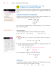### View a sample Math in Focus lesson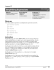### Grade level: 6 Subject: Mathematics Words Important for This Unit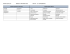### A.4 R E Domain of an Algebraic Expression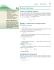### Interpreting Algebraic Expressions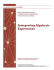### Algebraic Expressions - glencoe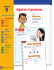### RATE CARD - Montreal Gazette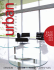### Page 1 of 3 Buncombe County Planning Board May 6, 2013 The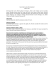### Unit 4 - Amazon Web Services### 33465_22 Acres on Smokey Hollow Rd_Dekorra_SBP_Sale_11TX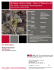### ShadowCon Talk (Graham Fletcher)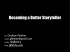### EXPRESSIONS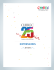### Document 1 - Property Partnership Australia